# 时间序列预测算法-DSP

Introduction for DSP Algorithm

Crane使用在数字信号处理（Digital Signal Processing）领域中常用的的离散傅里叶变换自相关函数等手段，识别、预测周期性的时间序列。

## 流程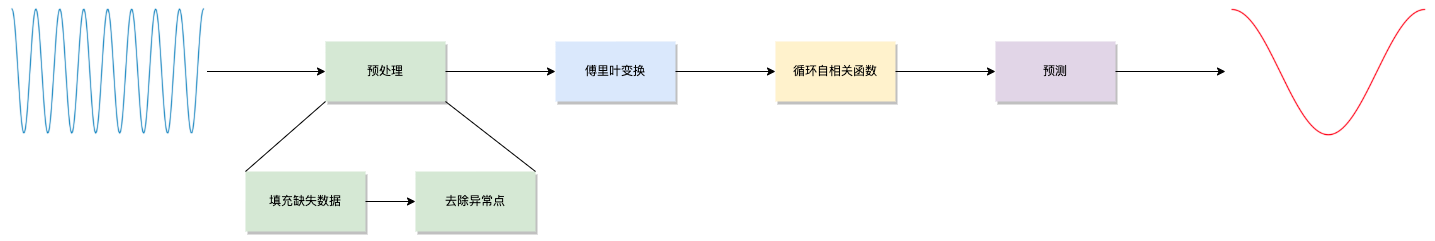### 预处理

#### 填充缺失数据#### 去除异常点

1. 监控系统用0值填充缺失的采样点；
2. 被监控组件由于自身的bug上报了错误的指标数据；
3. 应用启动时会消耗远超正常运行时的资源#### 离散傅里叶变换

$k = 0$对应信号的「直流分量」，对于周期没有影响，因此忽略。

$k$所对应的周期$$T = {N \over k} \bullet SampleInterval$$

Crane没有尝试发现任意长度的周期，而是指定几个固定的周期长度（$1d、7d$）去判断。并通过截取、填充的方式，保证序列的长度$N$为待检测周期$T$的整倍数，例如：$T=1d，N=3d；T=7d，N=14d$。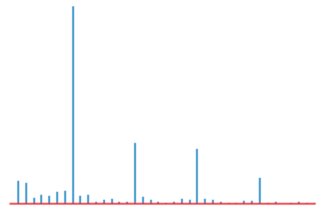1. 对原始序列$\vec x(n)$进行一个随机排列后得到序列$\vec x’(n)$，再对$\vec x’(n)$做FFT得到$\vec X’(k)$，令$P_{max} = argmax|\vec X’(k)|$。

2. 重复100次上述操作，得到100个$P_{max}$，取$P99$作为阈值$P_{threshold}$。

3. 对原始序列$\vec x(n)$做FFT得到$\vec X(f)$，遍历$k = 2, 3, …$，如果$P_k = |X(k)| > P_{threshold}$，则将$k$加入候选周期。

#### 循环自相关函数

Crane使用循环自相关函数（Circular ACF），先对长度为$N$的时间序列以$N$为周期做扩展，也就是在$…, [-N, -1], [N, 2N-1], …$区间上复制$\vec x(n)$，得到一个新的序列$\vec x’(n)$。 再依次计算将$\vec x’(n)$依次平移$k=1,2,3,…N/2$后的$\vec x’(n+k)$与$\vec x’(n)$的相关系数

$$r_k={\displaystyle\sum_{i=-k}^{N-k-1} (x_i-\mu)(x_{i+k}-\mu) \over \displaystyle\sum_{i=0}^{N-1} (x_i-\mu)^2}\ \ \ \mu: mean$$

Crane没有直接使用上面的定义去计算ACF，而是根据下面的公式，通过两次$(I)FFT$，从而能够在$O(nlogn)$的时间内完成ACF的计算。 $$\vec r = IFFT(|FFT({\vec x - \mu \over \sigma})|^2)\ \ \ \mu: mean,\ \sigma: standard\ deviation$$

ACF的图像如下所示，横轴代表信号平移的时间长度$k$；纵轴代表自相关系数$r_k$，反应了平移信号与原始信号的「相似」程度。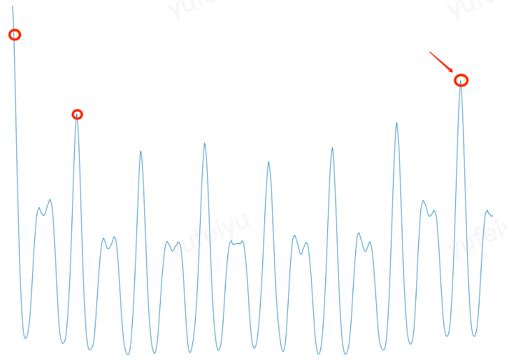Crane会依次验证每一个候选周期对应的自相关系数是否位于「山顶」上；并且选择对应「最高峰」的那个候选周期为整个时间序列的主周期（基波周期），并以此为基础进行预测。

Crane在两侧个各选取一段曲线，分别做线性回归，当回归后左、右的直线斜率分别大于、小于零时，则认为这个点是在一个「山顶」上。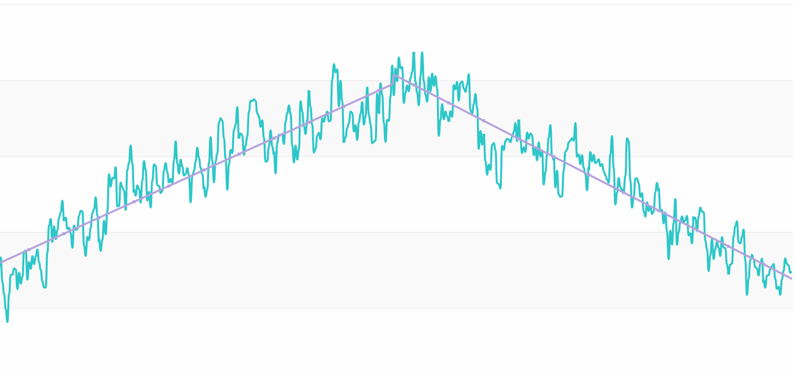#### 预测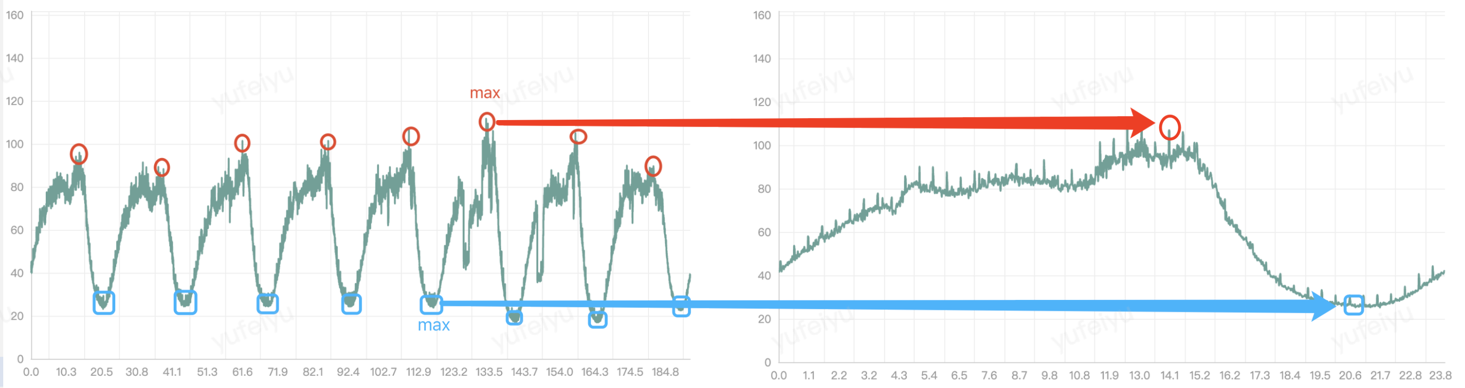fft

## 应用

Crane提供了TimeSeriesPrediction，通过这个CRD，用户可以对各种时间序列进行预测，例如工作负责的CPU利用率、应用的QPS等等。

apiVersion: prediction.crane.io/v1alpha1
kind: TimeSeriesPrediction
namespace: default
spec:
targetRef:
apiVersion: apps/v1
kind: Deployment
name: test
namespace: default
predictionWindowSeconds: 7200 # 提供未来7200秒（2小时）的预测数据。Crane会把预测数据写到status中。
predictionMetrics:
expression: 'sum (irate (container_cpu_usage_seconds_total{container!="",image!="",container!="POD",pod=~"^test-.*$"}[1m]))' # 获取历史监控数据的查询语句 algorithm: algorithmType: "dsp" # 指定dsp为预测算法 dsp: sampleInterval: "60s" # 监控数据的采样间隔为1分钟 historyLength: "15d" # 拉取过去15天的监控指标作为预测的依据 estimators: # 指定预测方式，包括'maxValue'和'fft'，每一类可以指定多个estimator，配置不同的参数，crane会选取一个拟合度最高的去产生预测结果。如果不指定的话，默认使用'fft'。 # maxValue: # - marginFraction: "0.1" fft: - marginFraction: "0.2" lowAmplitudeThreshold: "1.0" highFrequencyThreshold: "0.05" minNumOfSpectrumItems: 10 maxNumOfSpectrumItems: 20  上面示例中的一些dsp参数含义如下： maxValue marginFraction: 拟合出下一个周期的序列后，将每一个预测值乘以1 + marginFraction，例如marginFraction = 0.1,就是乘以1.1。marginFraction的作用是将预测数据进行一定比例的放大（或缩小）。 fft marginFraction: 拟合出下一个周期的序列后，将每一个预测值乘以1 + marginFraction，例如marginFraction = 0.1,就是乘以1.1。marginFraction的作用是将预测数据进行一定比例的放大（或缩小）。 lowAmplitudeThreshold: 频谱幅度下限，所有幅度低于这个下限的频率分量将被滤除。 highFrequencyThreshold: 频率上限，所有频率高于这个上限的频率分量将被滤除。单位Hz，例如如果想忽略长度小于1小时的周期分量，设置highFrequencyThreshold = 1/3600 minNumOfSpectrumItems: 至少保留频率分量的个数。 maxNumOfSpectrumItems：至多保留频率分量的个数。 简单来说，保留频率分量的数量越少、频率上限越低、频谱幅度下限越高，预测出来的曲线越光滑，但会丢失一些细节；反之，曲线毛刺越多，保留更多细节。 下面是对同一时段预测的两条曲线，蓝色、绿色的highFrequencyThreshold分别为$0.01$和$0.001$，蓝色曲线过滤掉了更多的高频分量，因此更为平滑。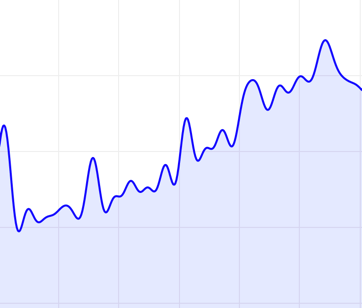并没有一套参数配置适合所有的时间序列，通常需要根据应用指标的特点，去调整算法参数，以期获得最佳的预测效果。 Crane提供了一个web接口，使用者可以在调整参数后，直观的看到预测效果，使用步骤如下： 1. 修改TimeSeriesPrediction中的estimators的参数。 2. 访问craned http server的api/prediction/debug/<namespace>/<timeseries prediction name>，查看参数效果（如下图）。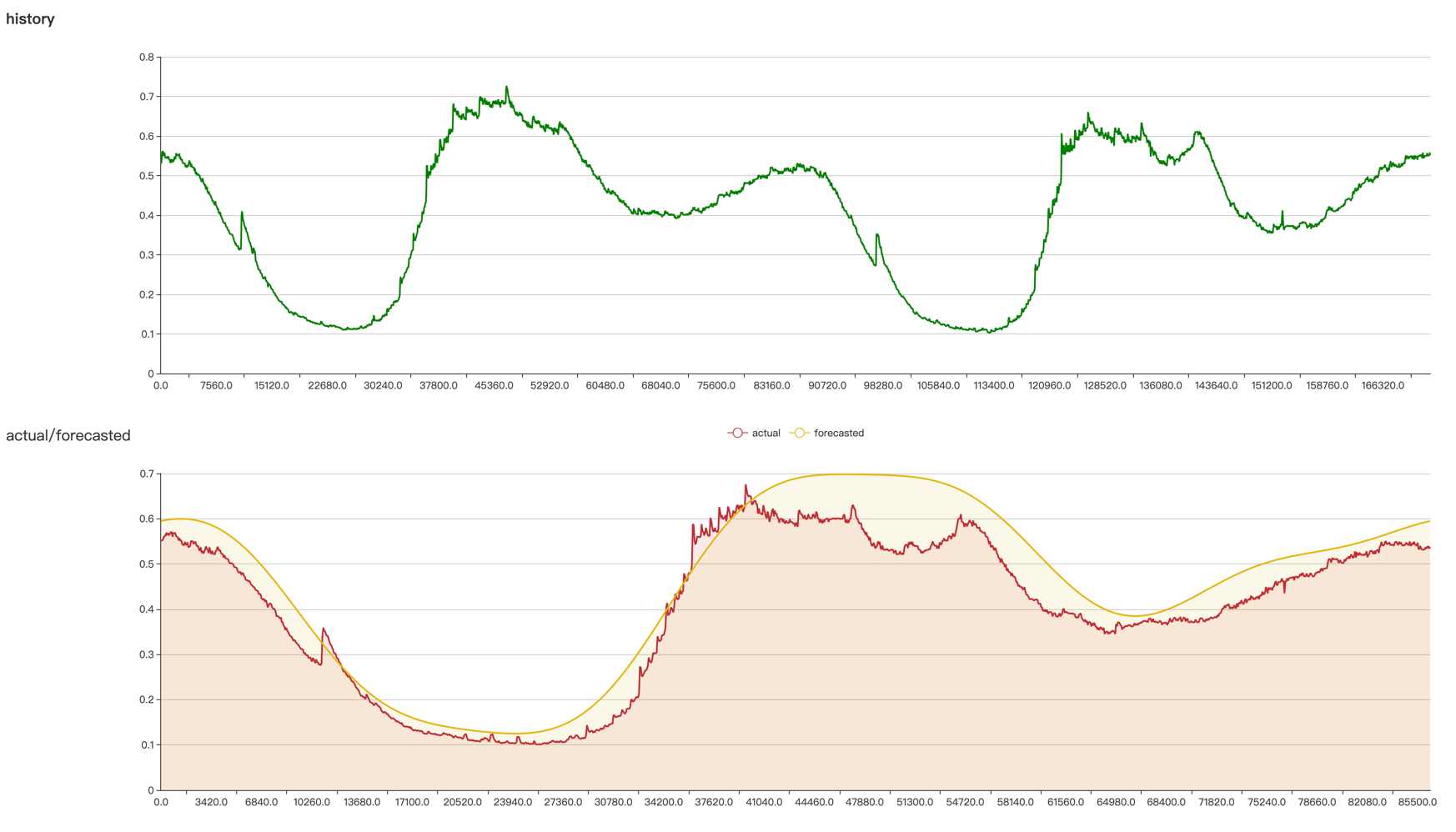上述步骤可多次执行，直到得到满意的预测效果。 通过port-forward进行本地调试 craned http server的端口通过craned启动参数--server-bind-port设置，默认为8082 打开终端， $kubectl -n crane-system port-forward service/craned 8082:8082